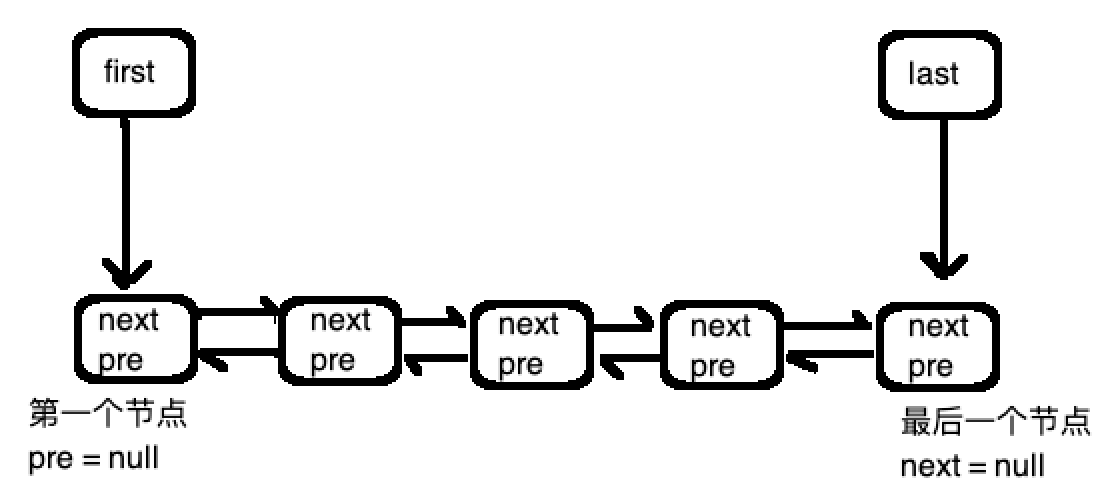public class QLinkedList<T> {
private QNode<T> first; //指向头节点
private QNode<T> last;  //指向尾节点

private int size;    //节点的个数

//节点类
public static class QNode<T> {
T value;        //数据
QNode<T> pre;   //指向上一个节点
QNode<T> next;  //指向下一个节点

public QNode(QNode<T> pre, QNode<T> next, T value) {
this.pre = pre;     //节点的上一个指向
this.next = next;   //节点的下一个指向
this.value = value; //存放的数据
}
}

//默认是一个空狼链表，first,last都为null, 节点个数为0
first = null;
last = null;
size = 0;
}

//默认添加到尾
}

//添加到头部
if (first == null && last == null) {
QNode<T> node = new QNode<>(null, null, e);
first = node;
last = node;
} else {
QNode<T> node = new QNode<>(null, first, e);
first.pre = node;
}

size++;
}

//添加到尾部,我们默认添加的都是不为null的数据
if (e == null) {
throw new RuntimeException("e == null");
}

//1 链表还是空的时候
if (size == 0) {

//1.1 新建一个节点，pre,next都为null
QNode<T> node = new QNode(null, null, e);

//1.2 只有一个节点，first,last都指向null
first = node;
last = node;

//2 链表不为空
} else {

//2.1 新建一个节点，pre指向last最后一个节点，next为null(因为是最后一个节点)
QNode<T> node = new QNode<>(last, null, e);

//2.2 同时之前的最后一节点的next 指向新建的node节点
last.next = node;

//2.3 然后移动last，让last指向最后一个节点
last = node;
}

//添加一个节点后，别忘了节点的总数加 1
size++;
}

// position 从 0 开始
// 这里面有个小技巧，可以先判断一下 position 是大于 size/2 还是小于 size/2
// 如果 position > size / 2 , 说明position是在链表的后半段，我们可以从last开始往前遍历
// 如果 position < size / 2, 说明position是在链表的前半段，我们可以从first开始往后遍历
// 这样效率会高许多，这也是双链表的意义所在，我们这里就不这样做了。直接从前往后遍历
// 读者可以自己实现，以加深对链表的理解
public T get(int position) {
// 不合法的position直接抛异常，让开发者直接定位问题
if (position < 0 || position > size - 1) {
throw new RuntimeException("invalid position");
}

// 如果链表为空，直接返回null
if (size == 0) {
return null;
}

// 如果链表只有一个节点，直接返回
// 因为position合法性在前面已经验证过
// 所以在这里面不用验证，一定是0
if(size == 1){
return first.value;
}

// 注意这个新建的 p 节点，p.next 指向的是 first
// 这是为了下面的循环，保证 i == 0 的时候，p 指向第一个节点
QNode<T> p = new QNode<>(null, first, null);
for (int i = 0; i <= position; i++) {
p = p.next;
}

//如果找到了，就返回value
if (p != null) {
return p.value;
}

//否则返回 null
return null;
}

// 返回链表的节点总个数
// 注意first和last节点只是帮助我们方便操作的
// size可不包括first,last
public int size() {
return size;
}

// 删除一个元素，这里传的参数是 T e ，我们也可以传position进行删除，这里就不作演示了
// 可以先调用上面的get()方法，返回对应的值，再调用此方法
// 读者可以自己实现
public T remove(T e) {
//1 不合法，抛异常
if (e == null) {
throw new RuntimeException("e == null");
}

//2 链表为空，返回 null
if (size == 0) {
return null;
}

//2 如果链表只有一个节点
if (size == 1) {
QNode<T> node = first;

//3 如果相等，删除节点 size-- ,并把first,last赋值为null
if(e == node.value || e.equals(node.value)){
first = last = null;
size--;
return node.value;
}else {
//4 不相等，返回null
return null;
}
}

// 如果链表大于1个节点，我们从前往后找value等于e的节点
// 1 查找， 和get()方法一样，注意p的next指向first
QNode<T> p = new QNode<>(null, first, null);
boolean find = false;
for (int i = 0; i < size; i++) {
p = p.next;

if (p != null && (e == p.value || e.equals(p.value))) {
find = true;
break;
}
}

// 2 如果找到了
if (find) {
// 2.1 如果找到的节点是最后一个节点
// 删除的是最后一个
if (p.next == null) {

//2.2 改变last的值，指向p的前一个节点
last = p.pre;

//2.3 p的前一个节点，变成了最后一个节点，所以，前一个节点的next值赋值为null
p.pre.next = null;

//2.4 把p.pre赋值为null,已经没有用了
p.pre = null;

//2.5 别忘了节点个数减1
size--;

//2.6 返回删除的节点的value
return p.value;

//3.1 如果删除的是第一个节点（p.pre == null就表明是第一个节点）
} else if (p.pre == null) {

//3.2 改变first的指向，指向p的下一个节点
first = p.next;

//3.3 p的下一个节点变成了第一个节点，需要把p的下一个节点的pre指向为null
p.next.pre = null;

//3.4 p.next没有用了
p.next = null;

//3.5 别忘了节点个数减1
size--;

//3.6 返回删除的节点的value
return p.value;

// 4 如果删除的不是第一个也不是最后一个，是中间的某一个，这种情况最简单
} else {

//4.1 p的上一个节点的next需要指向p的下一个节点
p.pre.next = p.next;

//4.2 p 的下一个节点的pre需要指向p的上一个节点
p.next.pre = p.pre;

//4.3 此时p无用了，把p的pre,next赋值为null
//这时候不需要调整first,last的位置
p.pre = null;
p.next = null;

//4.4 别忘了节点个数减1
size--;

//4.5 返回删除的节点的value
return p.value;
}
}

//没有找到与e相等的节点，直接返回null
return null;
}

}

   public static void main(String[] args) {

System.out.println(list.size);
for (int i = 0; i < list.size; i++) {
System.out.println(list.get(i));
}

System.out.println("===================");

System.out.println(list.remove("two"));
System.out.println(list.size);
for (int i = 0; i < list.size; i++) {
System.out.println(list.get(i));
}
}

4
one
two
three
four
===================
two
3
one
three
four